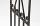Triangle SSA

Construct a triangle ABC if |AB| = 5cm va = 3cm, CAB = 50 °. It is to create the analysis and construction steps.

Result

a =  3.836 cm

Solution:

Try calculation via our triangle calculator.

Leave us a comment of example and its solution (i.e. if it is still somewhat unclear...):Be the first to comment!Next similar examples:

1. Triangle ABCConstruct a triangle ABC is is given c = 60mm hc = 40 mm and b = 48 mm analysis procedure steps construction
2. ConstructConstruct a triangle ABC inscribed circle has a radius r = 2 cm, the angle alpha = 50 degrees = 8 cm. Make a sketch, analysis, construction and description.
3. Ruler and compassUse a ruler and compass to construct a triangle ABC with AB 5cm BAC 60° and ACB 45°.
4. Construct 1Construct a triangle ABC, a = 7 cm, b = 9 cm with right angle at C, construct the axis of all three sides. Measure the length of side c (and write).
5. Right triangleDraw a right triangle ABC if |AB| = 5 cm |BC| = 3 cm, |AC| = 4 cm. Draw Thales circle above the hypotenuse of the triangle ABC.
6. Find the 9Find the missing angle in the triangle and then name triangle. Angles are: 95, 2x+15, x+3
7. Inscribed triangleTo a circle is inscribed triangle so that the it's vertexes divide circle into 3 arcs. The length of the arcs are in the ratio 2:3:7. Determine the interior angles of a triangle.
8. AnglesIn the triangle ABC, the ratio of angles is: a:b = 4: 5. The angle c is 36°. How big are the angles a, b?
9. 30-60-90The longer leg of a 30°-60°-90° triangle measures 5. What is the length of the shorter leg?
10. Internal anglesFind the internal angles of the triangle ABC if the angle at the vertex C is twice the angle at the B and the angle at the vertex B is 4 degrees smaller than the angle at the vertex A.
11. Center traverseIt is true that the middle traverse bisects the triangle?
12. Regular octagonDraw the regular octagon ABCDEFGH inscribed with the circle k (S; r = 2.5 cm). Select point S' so that |SS'| = 4.5 cm. Draw S (S '): ABCDEFGH - A'B'C'D'E'F'G'H'.
13. SlopeFind the slope of the line: x=t and y=1+t.
14. The ballThe ball was discounted by 10 percent and then again by 30 percent. How many percent of the original price is now?
15. TreesAlong the road were planted 250 trees of two types. Cherry for 60 CZK apiece and apple 50 CZK apiece. The entire plantation cost 12,800 CZK. How many was cherries and apples?
16. Two numbersWe have two numbers. Their sum is 140. One-fifth of the first number is equal to half the second number. Determine those unknown numbers.
17. PersonsPersons surveyed:100 with result: Volleyball=15% Baseball=9% Sepak Takraw=8% Pingpong=8% Basketball=60% Find the average how many like Basketball and Volleyball. Please show your solution.